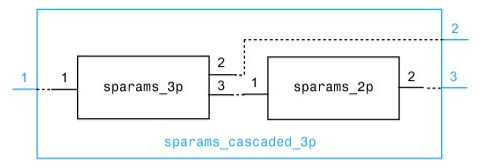Combine S-parameters to form cascade network

## Syntax

``````s_params= cascadesparams(s1_params,s2_params,...,sk_params)``````
``````s_params= cascadesparams(___,Kconn)``````
``````hs= cascadesparams(hs1,hs2,...,hsk)``````

## Description

example

``````s_params= cascadesparams(s1_params,s2_params,...,sk_params)``` cascades the scattering parameters (S-parameters) of K input networks described by the S-parameters. Each input network must be a 2N-port network described by a 2N-by-2N-by-M array of S-parameters for M frequency points. All networks must have the same reference impedance. NoteThe `cascadesparams `function uses ABCD-parameters. Alternatively, one can use S-parameters and ABCD-parameters (or T-parameters) to cascade S-parameters together by hand (assuming identical frequencies) ```
``````s_params= cascadesparams(___,Kconn)``` creates the cascaded networks based on the number of cascaded connections between the networks specified by `Kconn`. Use this option with the input arguments in the previous syntax.```
``````hs= cascadesparams(hs1,hs2,...,hsk)``` cascades K S-parameter objects to create a cascade network. The function checks that the impedance and frequencies of each object is equal and that the parameters of each object contain 2N-by-2N-by-M array of S-parameters for M frequency points. ```

## Examples

collapse all

Assemble a 2-port cascaded network from two sets of 2-port S-parameters operating at 2 GHz and at 2.1 GHz.

Create two sets of 2-port S-parameters.

```ckt1 = read(rfckt.amplifier,'default.s2p'); ckt2 = read(rfckt.passive,'passive.s2p'); freq = [2e9 2.1e9]; analyze(ckt1,freq); analyze(ckt2,freq); sparams_2p_1 = ckt1.AnalyzedResult.S_Parameters; sparams_2p_2 = ckt2.AnalyzedResult.S_Parameters;```

`sparams_cascaded_2p = cascadesparams(sparams_2p_1,sparams_2p_2)`
```sparams_cascaded_2p = sparams_cascaded_2p(:,:,1) = -0.4332 + 0.5779i 0.0081 - 0.0120i 2.6434 + 1.2880i 0.5204 - 0.5918i sparams_cascaded_2p(:,:,2) = -0.1271 + 0.3464i -0.0004 - 0.0211i 3.8700 - 0.6547i 0.4458 - 0.6250i ```

Assemble a 3-port cascaded network from a set of 3-port S-parameters and a set of 2-port S-parameters.Create one set of 3-port S-parameters and one set of 2-port S-parameters.

```ckt1 = read(rfckt.passive,'default.s3p'); ckt2 = read(rfckt.amplifier,'default.s2p'); freq = [2e9 2.1e9]; a1 = analyze(ckt1,freq); a2 = analyze(ckt2,freq); sparams_3p = sparameters(a1); sparams_2p = sparameters(a2);```

Cascade the two sets by connecting one port between them.

```Kconn = 1; sparams_cascaded_3p = cascadesparams(sparams_3p,sparams_2p,Kconn)```
```sparams_cascaded_3p = sparameters: S-parameters object NumPorts: 3 Frequencies: [2x1 double] Parameters: [3x3x2 double] Impedance: 50.0000 + 0.0000i rfparam(obj,i,j) returns S-parameter Sij ```

Assemble a 3-port cascaded network from a set of 3-port S-parameters and a set of 2-port S-parameters, connecting the second port of the 3-port network to the first port of the 2-port.```ckt1 = read(rfckt.passive,'default.s3p'); ckt2 = read(rfckt.amplifier,'default.s2p'); freq = [2e9 2.1e9]; a1 = analyze(ckt1,freq); a2 = analyze(ckt2,freq); sparams_3p = sparameters(a1); sparams_2p = sparameters(a2);```

Reorder the second and third ports of the 3-port network

`sparams_3p_2 = snp2smp(sparams_3p,[1 3 2]);`

Cascade the two sets by connecting one port between them

```Kconn = 1; sparams_cascaded_3p_2 = cascadesparams(sparams_3p_2,... sparams_2p,Kconn)```
```sparams_cascaded_3p_2 = sparameters: S-parameters object NumPorts: 3 Frequencies: [2x1 double] Parameters: [3x3x2 double] Impedance: 50.0000 + 0.0000i rfparam(obj,i,j) returns S-parameter Sij ```

Assemble a 3-port cascaded network from a set of 3-port S-parameters and two sets of 2-port S-parameters.```ckt1 = read(rfckt.passive,'default.s3p'); ckt2 = read(rfckt.amplifier,'default.s2p'); ckt3 = read(rfckt.passive,'passive.s2p'); freq = [2e9 2.1e9]; a1 = analyze(ckt1,freq); a2 = analyze(ckt2,freq); a3 = analyze(ckt3,freq); sparams_3p = sparameters(a1); sparams_2p_1 = sparameters(a2); sparams_2p_2 = sparameters(a3);```

Connect one port between each set of adjacent networks.

```Kconn = [1 1]; sparams_cascaded_3p_3 = cascadesparams(sparams_3p,... sparams_2p_1,sparams_2p_2,Kconn)```
```sparams_cascaded_3p_3 = sparameters: S-parameters object NumPorts: 3 Frequencies: [2x1 double] Parameters: [3x3x2 double] Impedance: 50.0000 + 0.0000i rfparam(obj,i,j) returns S-parameter Sij ```

Assemble a 3-port cascaded network from a set of 3-port S-parameters and two sets of 2-port S-parameters, connecting the 3-port network to both 2-port networks.

```ckt1 = read(rfckt.passive,'default.s3p'); ckt2 = read(rfckt.amplifier,'default.s2p'); ckt3 = read(rfckt.passive,'passive.s2p'); freq = [2e9 2.1e9]; analyze(ckt1,freq); analyze(ckt2,freq); analyze(ckt3,freq); sparams_3p = ckt1.AnalyzedResult.S_Parameters; sparams_2p_1 = ckt2.AnalyzedResult.S_Parameters; sparams_2p_2 = ckt3.AnalyzedResult.S_Parameters;```

Cascade sparams_3p and sparams_2p_1 by connecting one port between them.

```Kconn = 1; sparams_cascaded_3p = cascadesparams(sparams_3p, ... sparams_2p_1, Kconn);```

Reorder the second and third ports of the 3-port network.

```sparams_cascaded_3p_3 = snp2smp(sparams_cascaded_3p, ... 50, [1 3 2]);```

Cascade sparams_3p and sparams_2p_2 by connecting one port between them.

```sparams_cascaded_3p_4 = cascadesparams(sparams_cascaded_3p_3, ... sparams_2p_2, Kconn)```
```sparams_cascaded_3p_4 = sparams_cascaded_3p_4(:,:,1) = 0.1724 - 0.9106i 0.0240 + 0.0134i 0.0104 + 0.0971i -3.7923 + 6.1234i -0.7168 - 0.6498i -0.5855 + 1.6475i 0.1214 + 0.0866i 0.0069 + 0.0090i 0.6289 - 0.6145i sparams_cascaded_3p_4(:,:,2) = -0.3014 - 0.6620i 0.0072 - 0.0255i -0.0162 + 0.1620i 6.3709 + 2.2809i -0.5349 + 0.3637i 1.4106 + 0.2587i 0.0254 + 0.1011i 0.0087 - 0.0075i 0.5477 - 0.6253i ```

```T = tparameters('passive.s2p'); freq = T.Frequencies; for i = 1:length(freq) Tcascade(:,:,i) = T.Parameters(:,:,i)*T.Parameters(:,:,i); end Tcasc = tparameters(Tcascade,freq); Scasc_T = sparameters(Tcasc); rfplot(Scasc_T)```Validate results using `cascadesparams`.

```S = sparameters(T); Scasc = cascadesparams(S,S); rfplot(Scasc)```## Input Arguments

collapse all

S-parameters data, specified as a complex 2N-by-2N-by-M array.

S-parameter object, specified as a `sparameters` function object.

Number of cascade connections, specified as a positive scalar or vector.

• If `Kconn` is a scalar, `cascadesparams` makes the same number of connections between each pair of consecutive networks.

• If `Kconn` is a vector, the `i`th element of `Kconn` specifies the number of connections between the `i`th and the `i+1`th networks.

collapse all

### Port Ordering

The function assumes that the port ordering of the network is given byBased on this ordering, the function connects ports `N + 1` through `2N` of the first network to ports `1` through `N` of the second network. Therefore, when you use this syntax:

• Each network has an even number of ports

• Every network in the cascade has the same number of ports.

To use this function for S-parameters with different port arrangements, use the `snp2smp` function to reorder the port indices before cascading the networks.

### Kconn

`cascadesparams` always connects the last `Kconn(i)` ports of the `i`th network and the first `Kconn(i)` ports of the `i+1`th network. The ports of the entire cascaded network represent the unconnected ports of each individual network, taken in order from the first network to the `n`th network.

Also, when you specify `Kconn`:

• Each network can have either an even or odd number of ports.

• Every network in the cascade can have a different number of ports.